# Data fields

Description of the calculation with data fields

## Work with data fields

This page describes the calculation with multidimensional data fields. For more information of definition and caltulation see the page Creating and Calculating lists and tables.

Basicly

• Two data fields can be operands of a mathematical expression

• The elements of the first array are calculated with the corresponding elements of the second array

• If the array size is different, the extra elements of the longer field are ignored

Example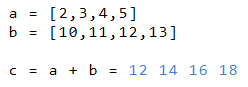In the next example, excessive elements of b are ignored.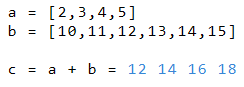In this example, the third line of b is ignored.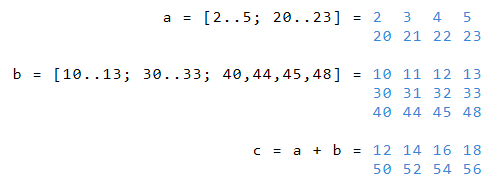This example shows the addition of a to rows 1 and 3 of variable b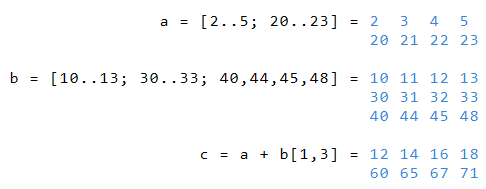## Create more-dimensional data arrays

In the above examples, each index of b is calculated with the corresponding index of b. Alternatively, RedCrab can compute arrays in which each index of a is computed with each index of b.

• The result is a multidimensional field of size a * b

• The empty brackets after c determine the result of the following calculation as a multidimensional array.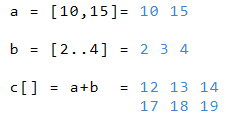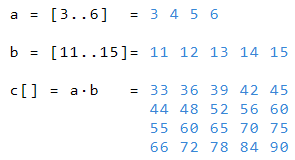The result of multiplying the one-dimensional array a and the two-dimensional array c is the three-dimensional array d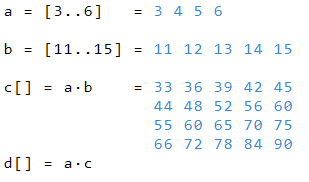• On the array level can be accessed via index

• The example below shows the four levels of the four-dimensional array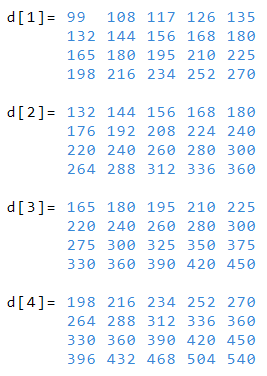• The following example shows how you can read the third column in the second level of d

• The apostrophe is the delimiter